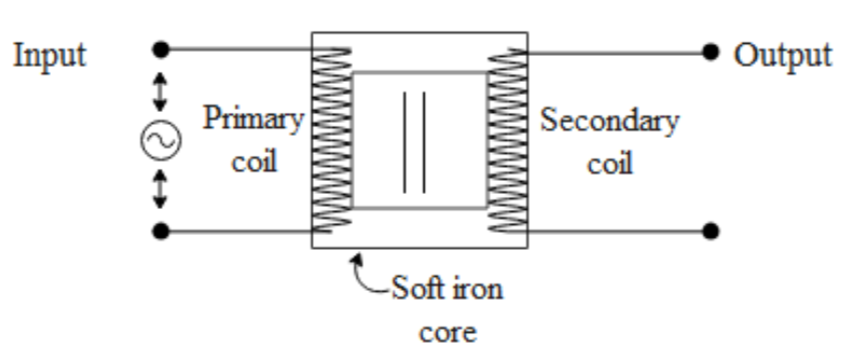QuestionAnswers

# The transformer works ona) AC only b) DC onlyc) Both AC and DCd) AC more efficiently than DCThe above figure represents a transformer wherein the primary and the secondary coils are mutually inducted. The emf is induced in a secondary coil only if there is a changing magnetic field generated by the primary coil. The field produced by a primary coil is given by i.e. $B={{\mu }_{\circ }}ni$ where ${{\mu }_{\circ }}$ is the permeability constant, n is the number of turns in the primary and i is the current through it. The field generated can induce emf in the secondary coil if and only if the current through it keeps on changing at any given instant of time. In a DC source, the current remains constant but in an AC source the current keeps on changing. Hence a transformer will only work on AC.